# 强势图解回文自动机

前置知识：

1、马拉车算法（大概吧，其实个人认为不学也没关系$qwq$）

2、$Trie$

关于回文自动机：

变量声明：

$1、ch[n][c]$：普通的字典树

$2、fail[n]$：$fail$指针，指向当前回文串的最长回文后缀，后面会详细介绍

$3、len[n]$：存放当前节点回文串的长度

$4、last$：最新添加的回文节点

$5、cnt$：总的节点个数

声明：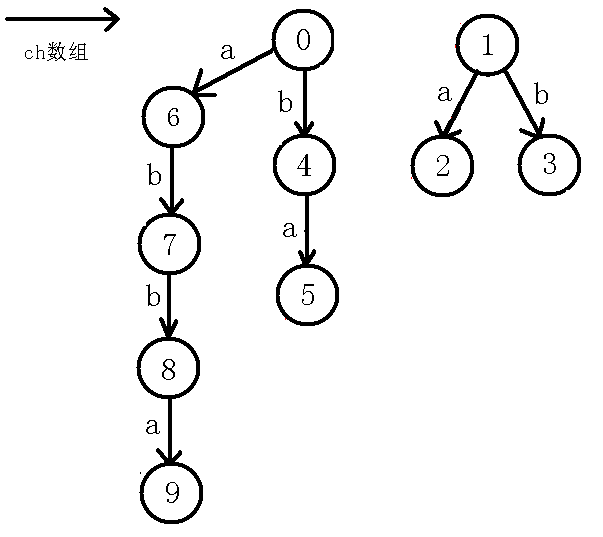$in=$'#'可以不是#，只要是不会出现的字符就行。

$fail=1$，$cnt=1$

1、找到最新建立的节点$last$，找到以$last$结尾的最长回文串的开头$-1$的位置，如果和当前位置字符相同的话（假设当前字符为$x$），说明在$last$这个回文串的基础上两边都有字符$x$，也就是说会有一个本质不同的回文串产生，否则跳$fail$指针，找到以$last$点结尾的最长回文后缀（不包括自身），最终找到$last$如果$last$节点没有$x$的转移，那么我们就让$cnt$自增$1$，即新建了一个节点，$len[cnt]=len[last]+2$因为我们找到$last$两边都是$x$字符，所以新的回文节点长度为原来的$+2$

2、如果$last$节点没有$x$的转移，那么我们就让$cnt$自增$1$，即新建了一个节点，$len[cnt]=len[last]+2$因为我们找到$last$两边都是$x$字符，所以新的回文节点长度为原来的$+2$，同时定义$j$为$fail[last]$，找到以当前节点的最长回文后缀，使$fail[cnt]$指向它。

3、更新$last$节点，继续插入下一个字符。

scanf("%d",&n);
scanf("%s",in+1);
in='#';
fail=1;len=-1;//初始化
for(int i=1;i<=n;i++)
{
int j;
while(in[i-len[last]-1]!=in[i])
last=fail[last];//匹配（后面会详细解说）
if(!ch[last][in[i]-'a'])//新建节点
{
len[++cnt]=len[last]+2;//长度比原来多2
j=fail[last];
while(in[i-len[j]-1]!=in[i])
j=fail[j];
fail[cnt]=ch[j][in[i]-'a'];
ch[last][in[i]-'a']=cnt;
}
last=ch[last][in[i]-'a'];
}
printf("%d",ans);


1、为什么要最长:

2、为什么要回文：

3、为什么要后缀：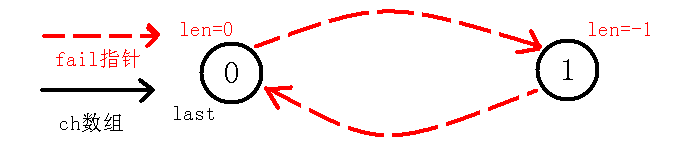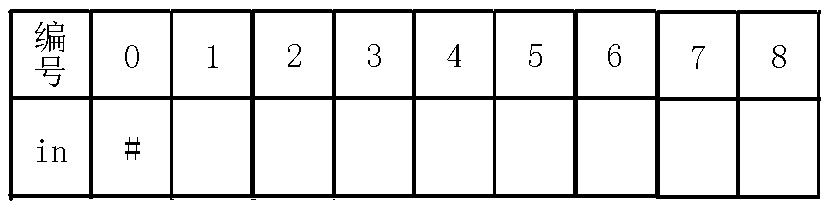1、插入字符$a$

$last$一开始为$0$，而$in[i-len[last]-1]!=in[i]$$in[1-0-1]!=in$，也就是说$in$与$in$不能构成回文串，那么我们就得缩小范围，$last$就跳$fail$指针到$1$节点，此时$in[i-len[last]-1]==in[i]$，也就是说$in==in$，也就是自己等于自己，因为我们把回文串的范围由$2$转为了$1$，所以现在回文串的长度也就是$1$，即$len[cnt]=len[last]+2$也就是$-1+2$，所以这也是$1$节点长度赋值为$-1$的好处，还保证了$1$的子树长度为奇数。此时$last$节点没有$a$的转移，我们就连一条边向$cnt$。即下图：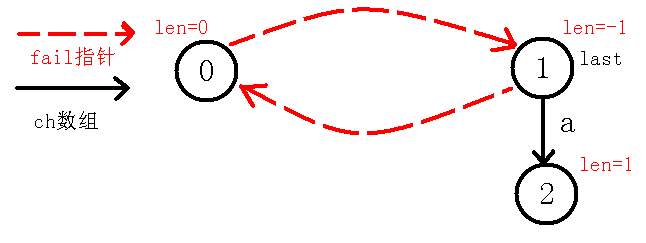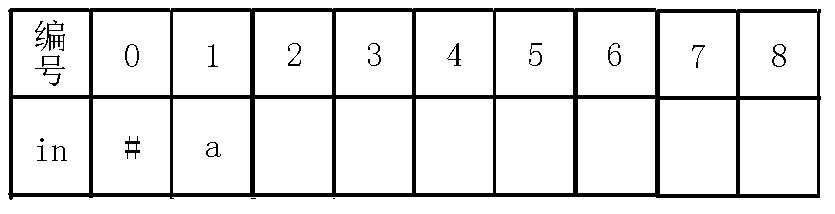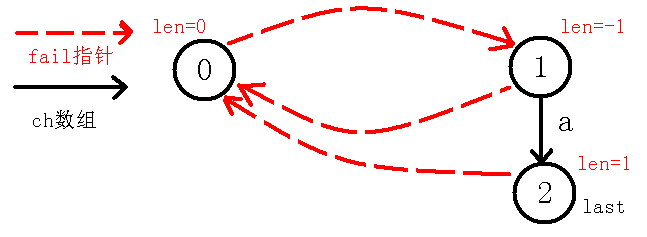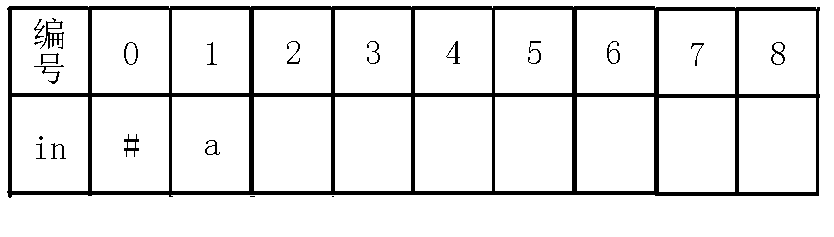2、插入$b$字符

$last$节点为$2$号节点，那么$in[i-len[last]-1]!=in[i]$，即$in!=in$，这个时候我们判断的其实是在$a$的两边是不是都是$b$，如果是的话那么肯定是回文串，可是这里并不匹配，所以$last$跳$fail$指针到$0$节点，但是我们发现$in[i-len[last]-1]!=in[i]$，即$in!=in$，这个时候我们判断的实质是，我们把回文串的范围由刚才的$3$个变为了$2$个，现在考虑是不是有两个$a$在一起构成回文串，可是还是失配了，所以我们再跳$fail$指针到$1$节点，而这时其实就是自己匹配自己了，也就是$in=in$，所以$len=len+2$，也就是1，因为这个回文串就$b$自身，长度自然为$1$，我们像上面一样连边：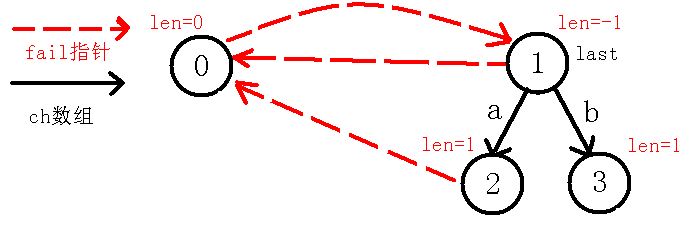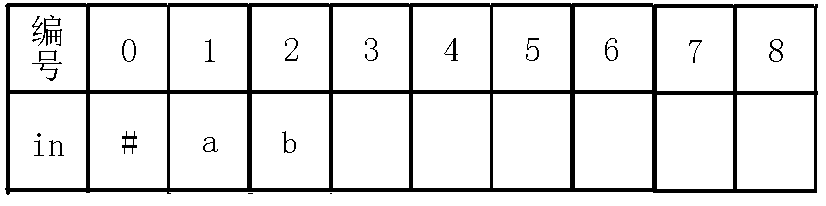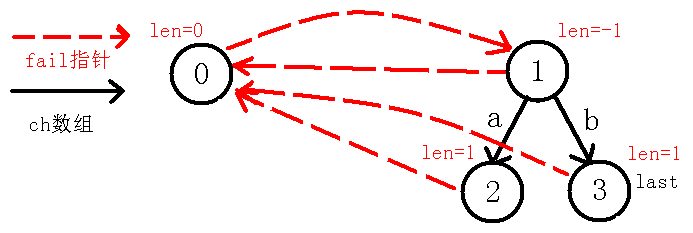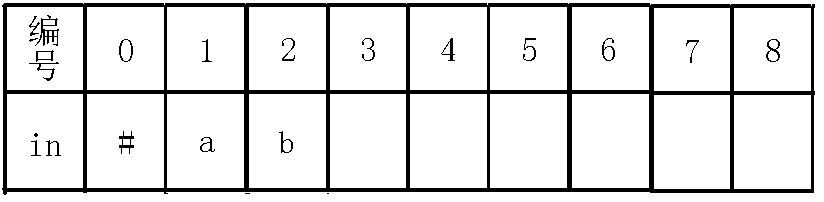3、插入字符$b$：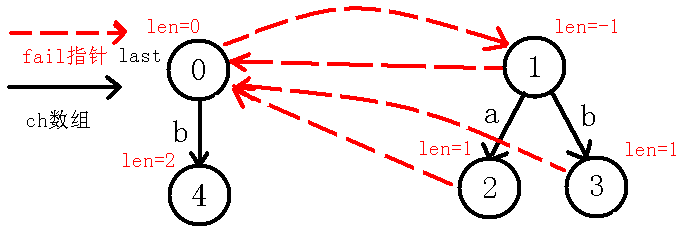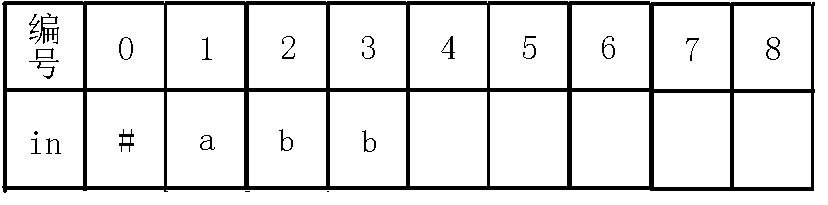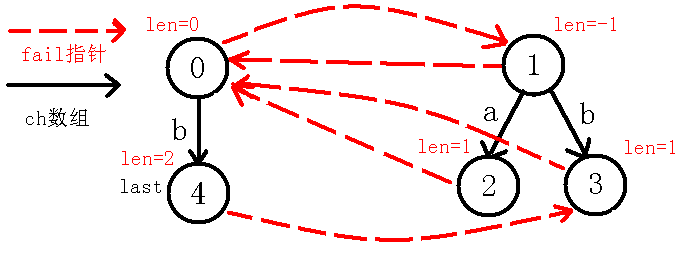4、插入$a$字符：

我们看着现在的$last$节点，可以看出$in[i-len[last]-1]=in[i]$，即$in=in$，也就是说，我们在$last$节点的基础上，即回文串$bb$的两边都找到了字符$a$，可以构成一个新的长度为$4$的回文串，而$last$节点没有$a$的转移，于是就新建节点，并连边，如下图：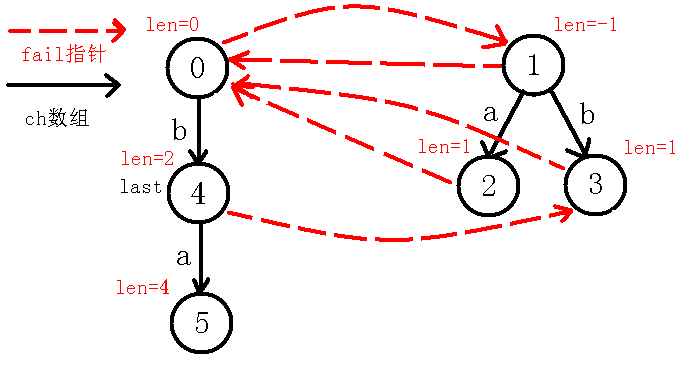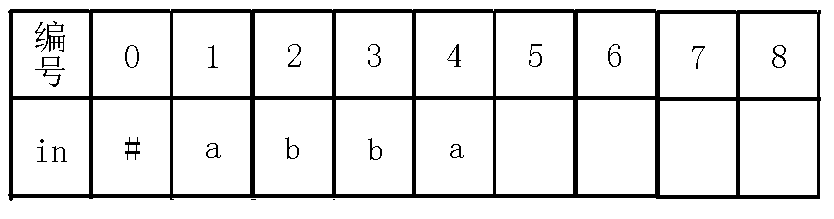【重点】：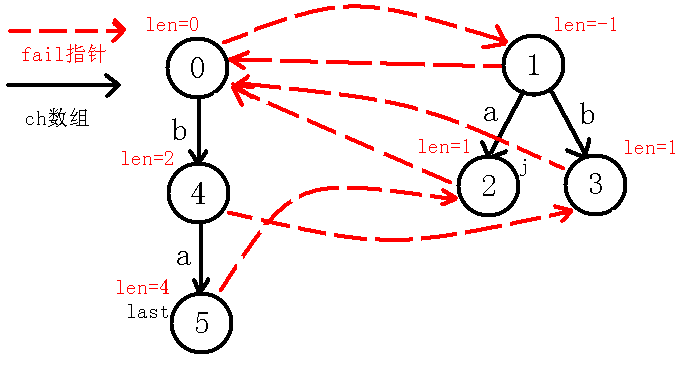5、插入$a$字符：

我们继续像上面一样模拟，看到$last$节点，我们发现$in[i-len[last]-1]!=in[i]$，也就是$in!=in$，我们这个操作实际上是找$abba$ 两端是否相同，如果相同则说明能够成新的回文串，然而失配了。。于是我们继续跳$fail$指针，到了$2$号节点，我们发现$in[i-len[last]-1]!=in[i]$，即$in!=in$，也就是$a$的两端相不相等，我们又发现不相等，于是又跳$fail$指针，到了$0$节点，我们发现此时$in[i-len[last]-1]=in[i]$，也就是$in=in$，说明我们找到了新的回文串$aa$，而$last$节点并没有$a$的转移（也就是以前没找到回文串$aa$ ），我们新加一条边到新的节点，如下图：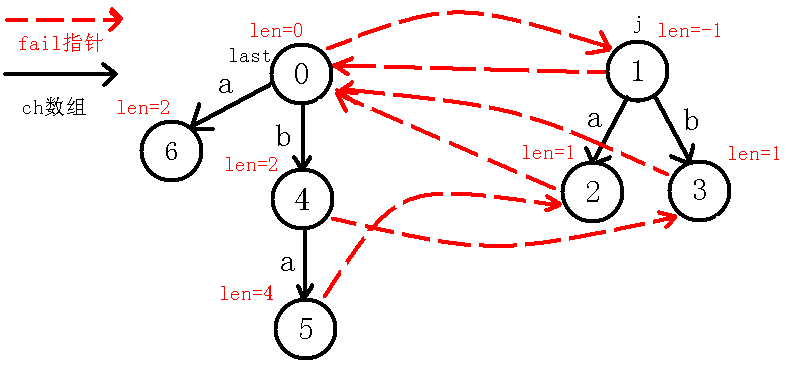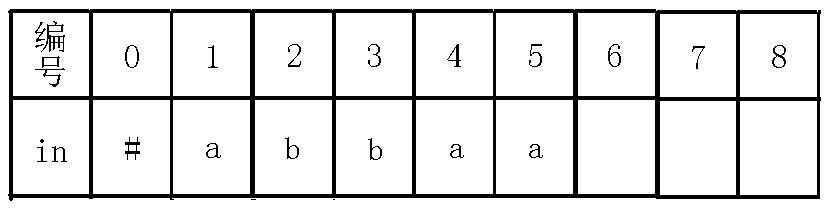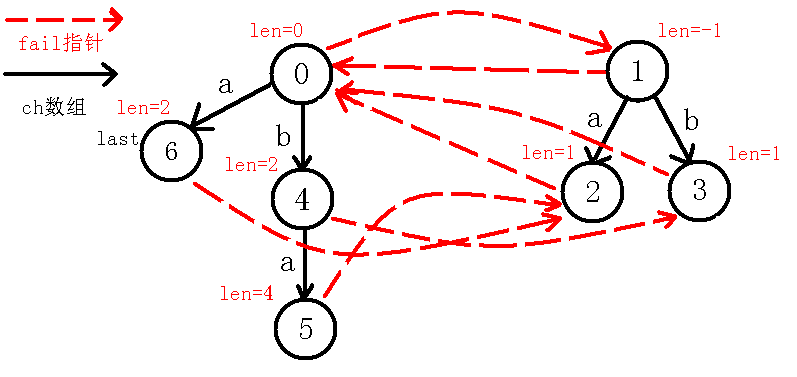（后文的插入就留给你们自己动手模拟了，本人就贴个图了，我觉得应该要给你们自己动手实战一下，其实是本人太懒$qwq$）

6、插入$b$字符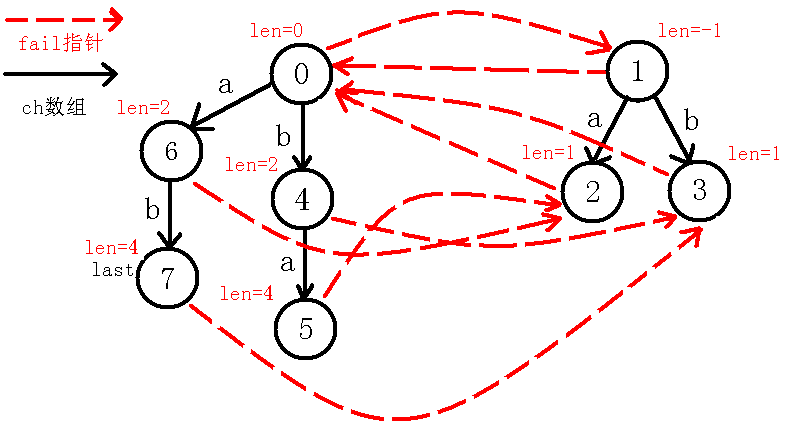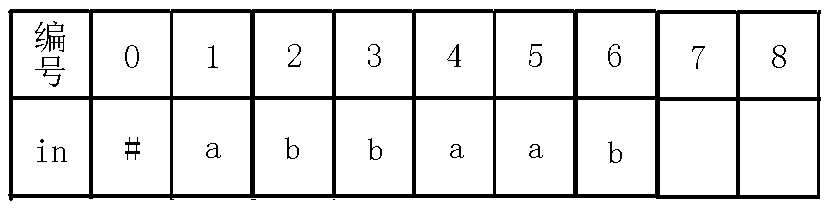7、插入$b$字符：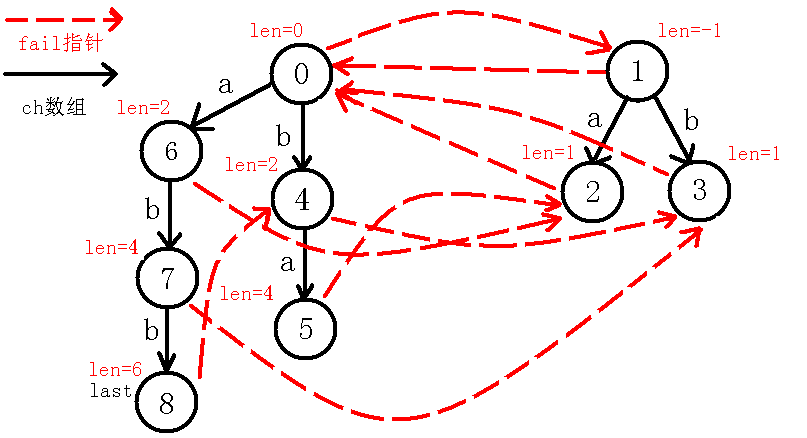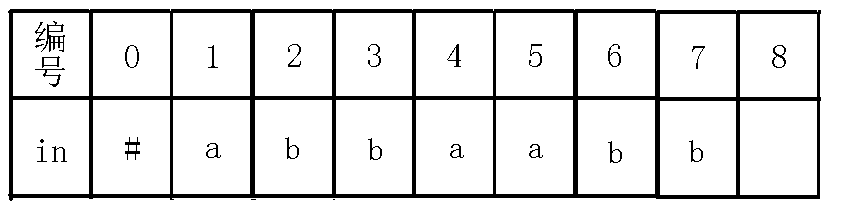8、插入$a$字符：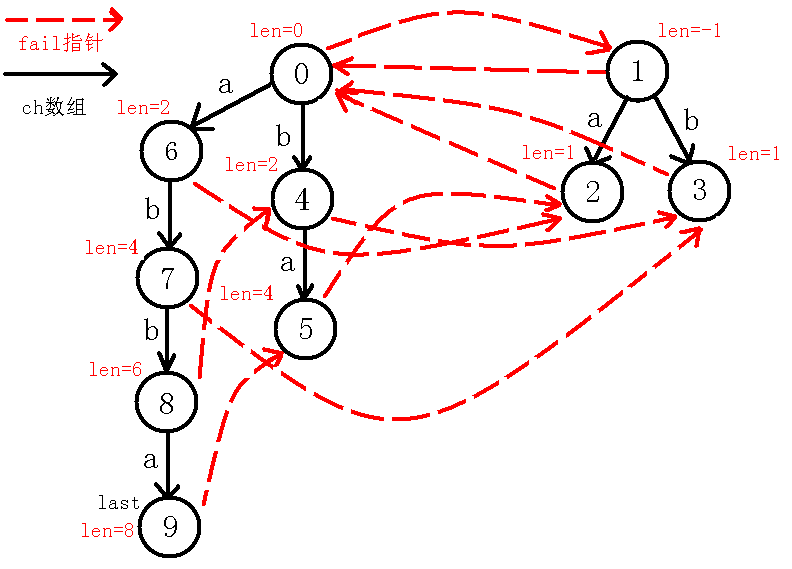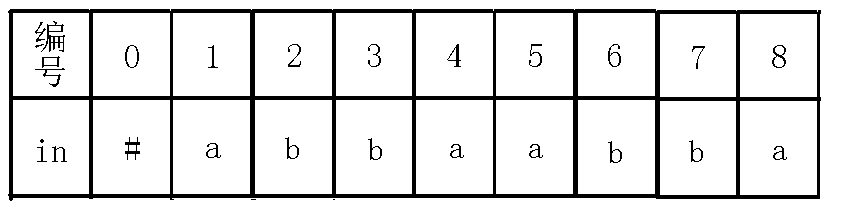posted @ 2018-12-08 10:19  模拟退火  阅读(1314)  评论(5编辑  收藏  举报